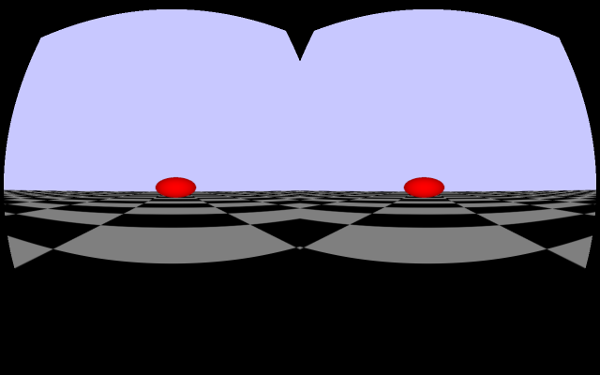## oculus experiment - processing sphere

I got the oculus rift DK1 to play with for a view days (thanks fake!!!!) so I started experimenting a little bit - here is my first processing sketch - a reminiscence on the 3D graphics I started with on my atari ST.

To run it you need the SimpleOculusRift library for processing by xohm.import SimpleOculusRift.*; SimpleOculusRift o;

``````void setup() {
size(1280, 800, OPENGL);
o = new SimpleOculusRift( this, SimpleOculusRift.RenderQuality_Middle );
o.setBknColor(200, 200, 255);
}

void draw() {
o.draw();
}

void onDrawScene(int eye) {
lights();
pushMatrix();
fill(255,0,0);
translate(0,0,-16);
sphere(1);
popMatrix();
for ( int y=0; y<40; y++) {
for ( int x=0; x<40; x++) {
fill( (x+y) % 2 == 0 ? 0 : 255 );
noStroke();
beginShape();
vertex( (x-20)*4.0+10, -2, (y-20)*4.0 );
vertex( (x-20)*4.0+10, -2, (y-19)*4.0 );
vertex( (x-19)*4.0+10, -2, (y-19)*4.0 );
vertex( (x-19)*4.0+10, -2, (y-20)*4.0 );
endShape();
}
}
}

void keyPressed() {